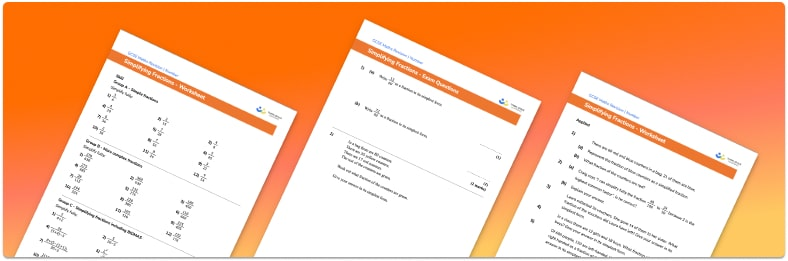# Simplifying Fractions Worksheet• Section 1 of the simplifying fractions worksheet contains 20+ skills-based simplifying fractions questions, in 3 groups to support differentiation
• Section 2 contains 3 applied simplifying fractions questions with a mix of word problems and deeper problem solving questions
• Section 3 contains 3 foundation and higher level GCSE exam style practice simplifying fractions questions
• An answer key and a mark scheme for all simplifying fractions questions are provided
• Questions follow variation theory with plenty of opportunities for students to work independently at their own level
• All worksheet pages and resource types are created by fully qualified expert secondary maths teachers
• Suitable for GCSE maths revision for AQA, OCR and Edexcel exam boards

• This field is for validation purposes and should be left unchanged.

You can unsubscribe at any time (each email we send will contain an easy way to unsubscribe). To find out more about how we use your data, see our privacy policy.

### Simplifying fractions at a glance

Sometimes we can simplify a fraction. This can make the fraction easier to understand. To simplify a fraction we look for common factors of the numerator and the denominator. We then divide both the numerator and the denominator by the same common factor to make them both smaller. We repeat this process until the numerator and denominator have no more common factors and the fraction is reduced to its lowest terms. A fraction is the division of two integers; a fraction that contains a decimal numerator or denominator must be simplified to two integers.

Looking forward, students can then progress to additional comparing fractions worksheets and other number worksheets, for example a rounding worksheet or percentageFor more teaching and learning support on Number our GCSE maths lessons provide step by step support for all GCSE maths concepts.

## Do you have KS4 students who need more focused attention to succeed at GCSE?There will be students in your class who require individual attention to help them succeed in their maths GCSEs. In a class of 30, it’s not always easy to provide.

Help your students feel confident with exam-style questions and the strategies they’ll need to answer them correctly with our dedicated GCSE maths revision programme.

Lessons are selected to provide support where each student needs it most, and specially-trained GCSE maths tutors adapt the pitch and pace of each lesson. This ensures a personalised revision programme that raises grades and boosts confidence.

Find out more